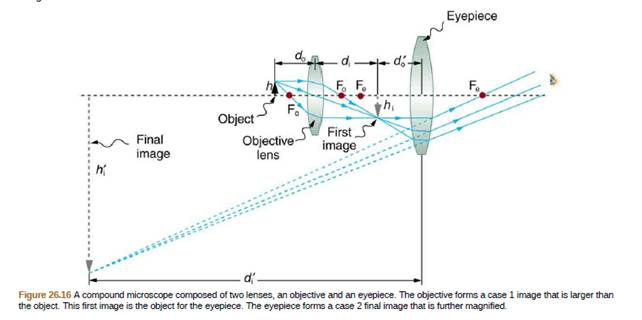Chapter 26, Problem 15CQ

Chapter
Section
Textbook Problem

The image produced by the microscope in Figure 25.16 cannot be projected. Could extra lenses or mirrors project if? Explain.To determine

Could extra lenses or mirrors project it?

Explanation

Introduction:

The compound microscope is an instrument for observing very small objects of the order of microns which is built on the basis of two convex lenses. One is called the objective lens and the other is called the eyepiece, which is often called the ocular. The image formed by this microscope is virtual and inverted, therefore based on those characteristics the image formed cannot be projected.

According to the sign conventions that are deduced from the equation of lenses and mirrors:

1f=1di+1do    (1)

And the magnification:

<

Still sussing out bartleby?

Check out a sample textbook solution.

See a sample solution

The Solution to Your Study Problems

Bartleby provides explanations to thousands of textbook problems written by our experts, many with advanced degrees!

Get Started

Find more solutions based on key concepts